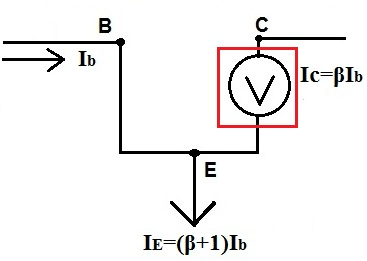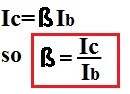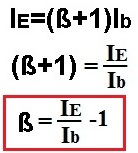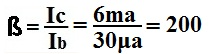﻿ How to Calculate β (Beta) of a Transistor# How to Calculate β (Beta) of a Transistorβ (beta), the gain or amplification factor of a transistor, normally is given when solving a circuit equation. However, if it is not given, it can be calculated if the currents, Ib (the base current) and either Ie ( the emitter current) or Ic (the collector current) are known.

If Ib and Ic are known, then β can be calculated by the formula:If Ib and Ie are known, then β can be calculate by the formula:Example

If Ic=6ma and Ib=30μa, then:Related Resources